# Lesson 1 Basics

The objective of this lesson is for you to get up and running with python. You will learn how to use the Google Colaboratory environment to write your python code and some basic coding principles.

Outline

• Coding in notebooks
• Using python as a calculator
• Operators
• Basic “built in” functions
• Documentation and getting help
• Summary
• Exercise 1.1
• Exercise 1.2
• Assignment 1

## 1.1 Working in Google Colab

Google Colab is interactive computing environment that enables users to author notebook documents that include:

• Live code
• Interactive widgets
• Plots
• Narrative text
• Equations
• Images
• Video

You can access your interactive python coding environment to create ipython notebooks at: https://colab.research.google.com/Figure 1.1: Google Colab - Landing Page

Once you sign in to Google Colab, you should be able to see a web page that looks like Figure 1.2.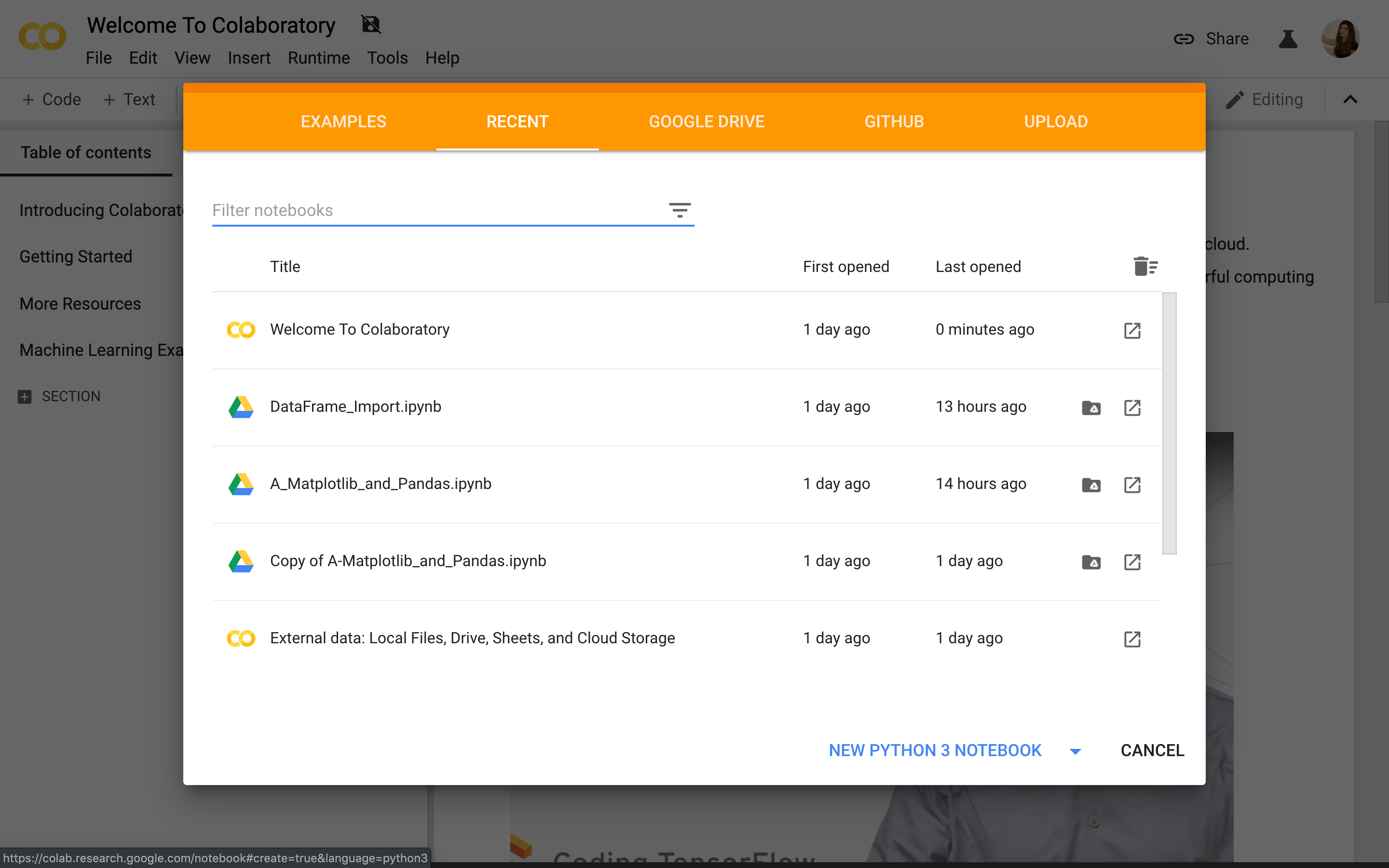Figure 1.2: Google Colab - Recent Notebooks Page

## 1.2 Coding in notebooks

In Google Colab, select NEW PYTHON 3 NOTEBOOK from the bottom of the Recent Notebooks screen to create a new ipython notebook. Notebooks are a way to program using python code. Think of a notebook as a simple text document that you can include python code and regular text. The python code you will write will serve as a set of instructions for the computer (i.e. Google Colab) to execute, see Figure 1.3.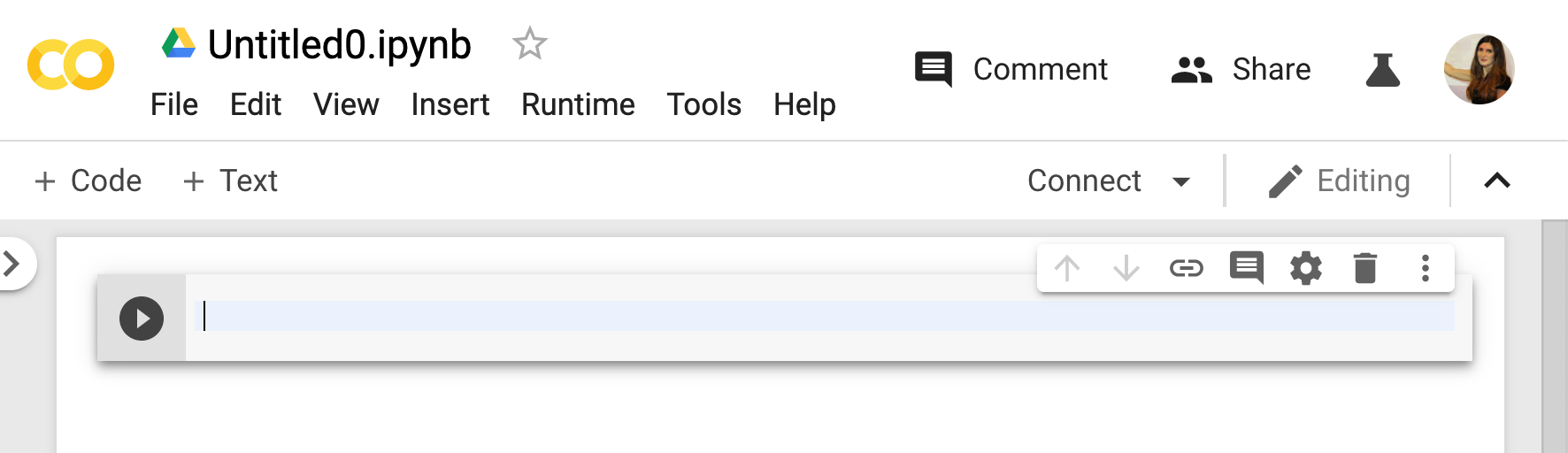Figure 1.3: Creating a new notebook

Rename your notebook to save it. Change the title from Untitled to Lesson01.ipynb by double clicking the notebook title, see Figure 1.4.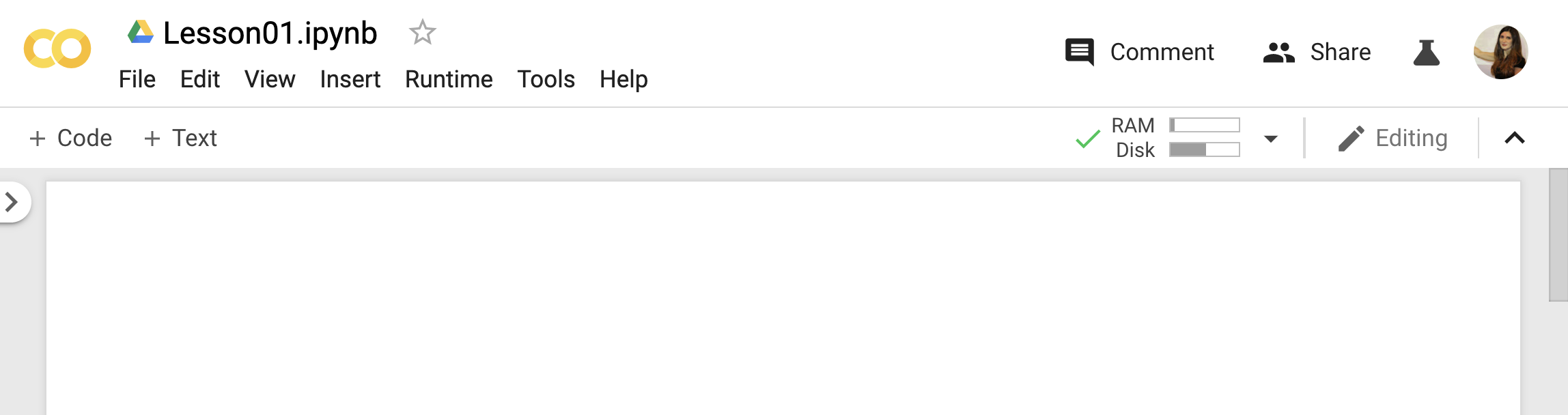Figure 1.4: Saving and naming your notebook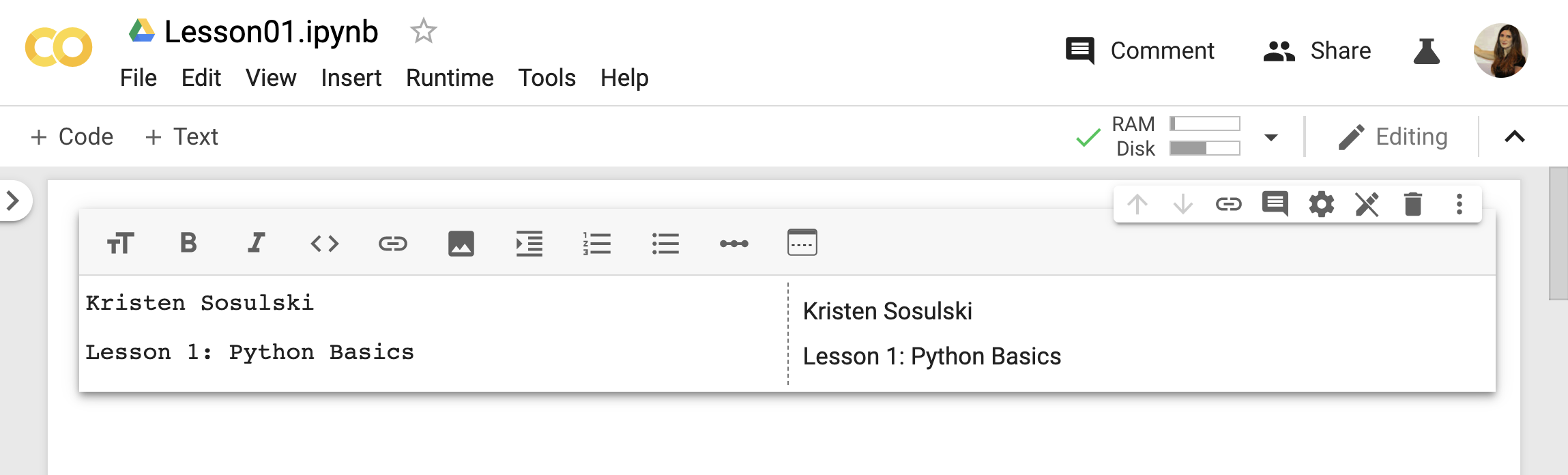Figure 1.5: Adding a text cell to a python notebook

Next, add a little `python` code. To do this, use the + Code option on the toolbar to add a code cell below your name.

Type the following:

``print("I'm using python to print text.")``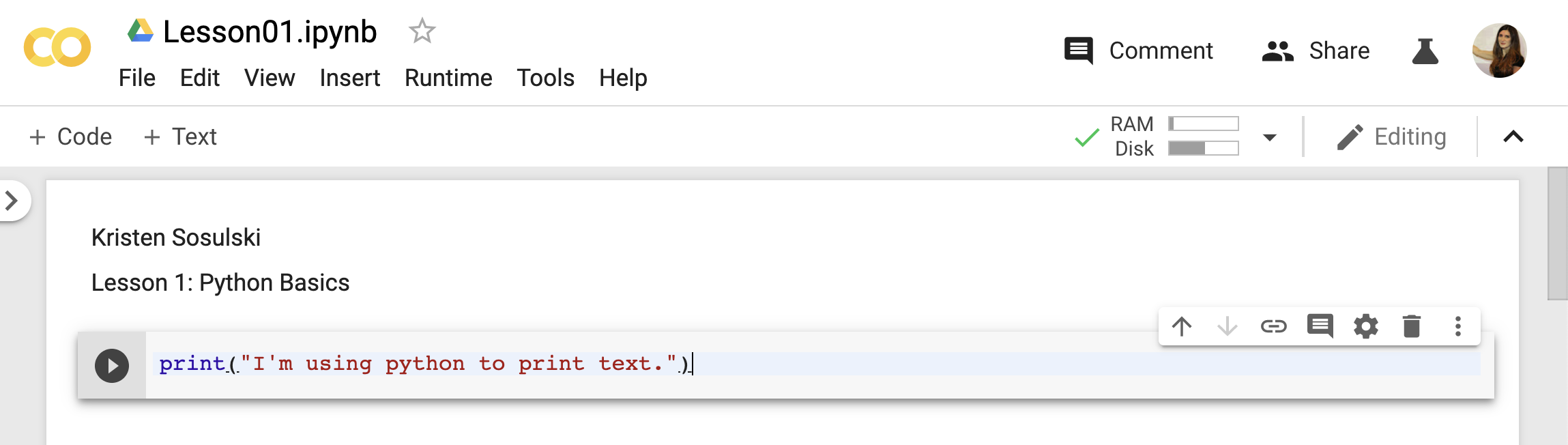Figure 1.6: Adding a code cell to a python notebook

Then, press the `Run` button. It looks a play button next to the code cell. This will execute the code that you just wrote, see Figure 1.7.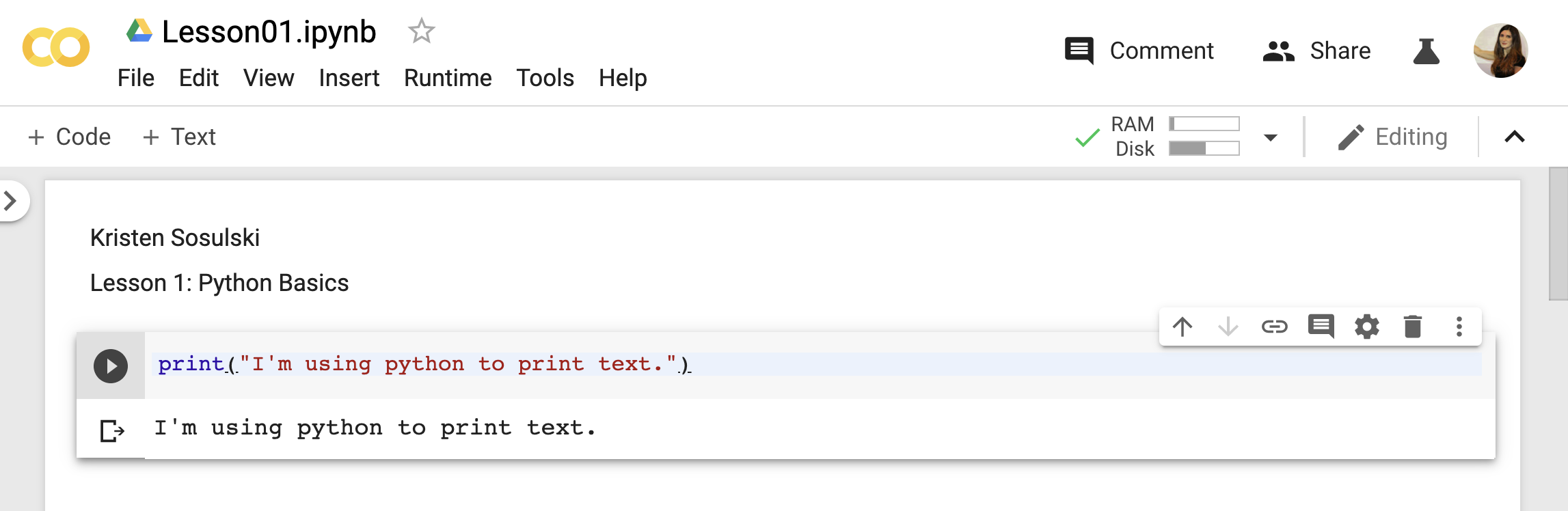Figure 1.7: Running code chunks

The code above is actual python code. You used a `function` named `print()` to display a `string` of text to the screen. The `input` was the code above and the output is:

``I'm using python to print text to the screen.``

You’ll be writing all your code in code cells within a notebook. For the first lesson, we will write one or two lines of code in each cell. These code chunks will allow us to cleasrly distinguish between the input and the output.

Type in the following code in a new code cell and run it.

``print(I'm using python to print text to the screen.)``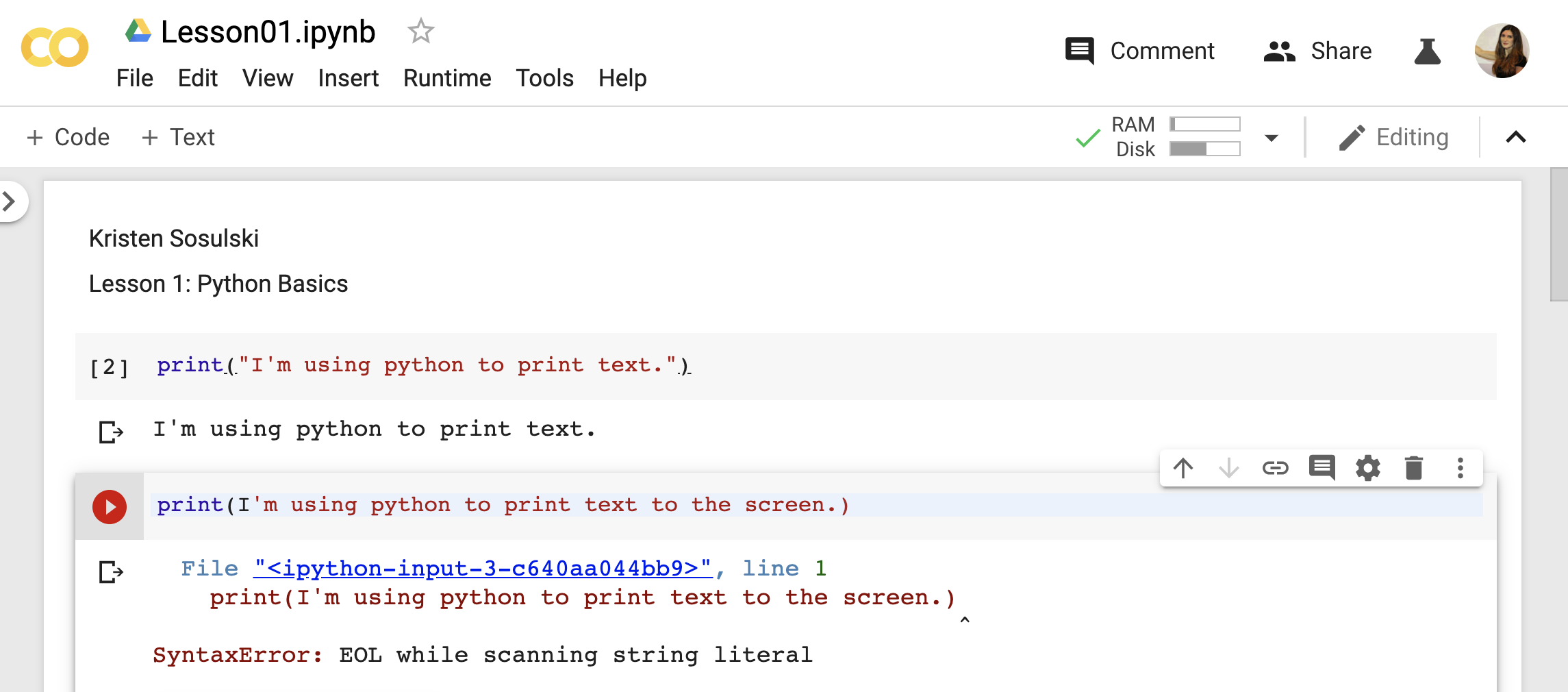Figure 1.8: Running code chunks

Did you notice that an error message was `returned` as the output?

`SyntaxError: EOL while scanning string literal`

This is called a `syntax error`. Syntax is the group of rules of a programming language. These rules are very important in order for the computer to know what you are trying to tell it using a language it can understand. These error messages are useful to catch our mistakes. Clearly, we forgot to put double quotes around `I'm using python to print text to the screen`. `EOL while scanning string literal` is one of most common syntax errors.

Another common syntax error is called `invalid syntax` or `NameError`. This error is shown usually when you misspell a function, such as `print()`. Let’s try to create a syntax error.

``prnt("This will cause a syntax error.")``Figure 1.9: Running code chunks

Misspelling the `print` function as `prnt` caused this error:

`NameError: name 'prnt' is not defined`

## 1.3 Using python as a calculator

We will use python just as we would a calculator. That is, we will use numbers and operators to perform calculations. The numbers and operators are the input and the result is the output. Python understands mathematical operations such as addition (`+`), subtraction (`-`), multiplication (`*`), and division(`/`). The symbols used by python to perform these operators are call operators. With these operators, we can use python as a calculator.

For example, if we wanted to add two numbers together we would simply type:`3 + 3` as our input and the result (i.e. the output) is `6`.

It’s easy to simply type in simple python expressions in a new cell in your notebook. For example, type 3+3 in a new code cell.

``3+3``
``6``

It’s important to note that incomplete expressions such as `3+` will return an error as shown below.

``3+``

`invalid syntax`

You can certainly include more than one line of code within a code cell. However, you will only see the result of the last line within the cell.

Input

``````3+3
9-2
993-42``````

Output

``951``

## 1.4 Operators

In the example above, we used the `+` operator to add two numbers together. This is called an arithmetic operator. There are many arithmetic operators used in python. The first five operators in Table 1.1 are used very frequently in python and throughout this course.

Operation Operator Example Input Example Output
Addition `+` `100 + 2` `102`
Subtraction `-` `93 - 3` `90`
Multiplication `*` `10 * 10` `100`
Division `/` `100 / 5` `20`
Integer Division `//` `65 // 10` `6`
Power `**` `8**2` `64`
Modulus (remainder for integer division) `%` `65 % 10` `5`

Table 1.1 Arithmetic Operators in Python

Order of operations

• When writing Python expressions, it is usually best to indicate the order of operations by using parentheses.

• If parentheses are not used in an expression, the computer will perform the operations in an order determined by the precedence rules.

Precedence rules (PEMDAS)

Parentheses have the highest precedence and can be used to force an expression to evaluate in the order you want. Since expressions in parentheses are evaluated first, `2 * (3-1)` is `4`, and `(1+1)**(5-2)` is `8`. You can also use parentheses to make an expression easier to read, as in `(minute * 100) / 60`, even if it doesn’t change the result. > - Exponentiation has the next highest precedence, so `2**1+1` is `3`, not `4`, and `3*1**3` is `3`, not `27`. > - Multiplication and Division have the same precedence, which is higher than Addition and Subtraction, which also have the same precedence. So `2*3-1` is `5`, not `4`, and `6+4/2` is `8`, not `5`.

Let’s try using some of these operators to help us solve simple problems.

1. What would be the total balance in the account at the end of the first month on your savings of `\$25,000` with a monthly interest rate is `1.99%`?

This involves using multiplication and addition.

``25000 * .0199 + 25000``
``25497.5``
1. Compute the hourly rate for an employee who works 40 hours per week at a monthly (4 weeks) salary of 21,400.
``21400/(40 * 4)``
``133.75``

We can use parentheses in python to specify the order of operations to ensure 21,400 is divided by the product of 40 * 4.

## 1.5 Basic built in functions

A built in function is a block of code that we can’t see from the python library. It only runs when it is called. You can pass data, known as parameters, into a function. A function can return data as a result. There are many built-in functions in python, see the Table 1.2 below.

Basic python functions

Absolute Value `abs( )` `abs(-21)` `21`
Maximum value `max()` `max(1,2,3,4,5)` `5`
Minimum value `min()` `min(1,2,3,4,5)` `1`
Print to the screen `print()` `print("Hello World!")` `Hello World!`
Round a number to a specified number of decimal places `round( )` `round(3.432,2)` `3.43`

Table 1.2 Basic python functions

Let’s try out these functions

### 1.5.1`abs()`

The absolute value of a real number x is the non-negative value of x without regard to its sign function. For example, absolute value of -33 is 33.

In python we write the absolute value |-33| as `abs(-33)`.

``abs(-33) ``
``33``

### 1.5.2`max()`

For a given sequence of numbers, you can find the maximum value. You can enter in a number sequence, separated by commas in between the parentheses of the `max()` function.

``max(2,3,4,5,6) # this sequence is called a tuple``
``6``

You can also enter (or pass in) a sequence of numbers using the square brackets `[ ]`. This creates different type of data. More on that in lesson 2.

``max([2,3,4,5,6]) # this sequence is called a list``
``6``

### 1.5.3`min()`

The `min()` function works similar to the max() function, except that the smallest value is returned.

Passing in a tuple

``min(2,3,-4,5,6) # this sequence is called a tuple``
``-4``

Passing in a list

``min([2,3,-4,5,6]) # this sequence is called a list``
``-4``

### 1.5.4`print()`

We’ve used the `print()` function to display text to the screen. Text is always passed into the `print()` as a string or `str`. The string in enclose in double quotes as shown below. We will learn more about strings in lesson 2.

``print("Printing a string to the screen.") ``
``Printing a string to the screen.``

In addition to printing strings, you can show the output of your computations to the screen. For example you can pass in 2+2. Here double quotes are not used. This is because we are adding numbers together, not printing text to the screen. The result of 4 will be returned.

``print(2+2) ``
``4``

You can see the print function prints the output of computations and strings. To print both the string and the computation in a single `print()` statement, you would separate each by a comma.

``print("The result is:", 2+2) ``
``The result is: 4``

### 1.5.5`round()`

You can round a number to specified number of decimal places using the `round()` function. In the example below, 4333.4222 is rounded to 2 decimal places. The first parameter is the number you wish to round and the second parameter is the number decimal places you want to round the first parameter.

``round(4333.4222, 2) ``
``4333.42``

It’s important to understand the parameters a function takes so you can code it properly. For example:

``round(4333.4222, 2,1) ``

This produces a `TypeError` with the following explanation:

`round() takes at most 2 arguments (3 given)`.

You can find the full list of built-in functions at: https://docs.python.org/3.7/library/functions.html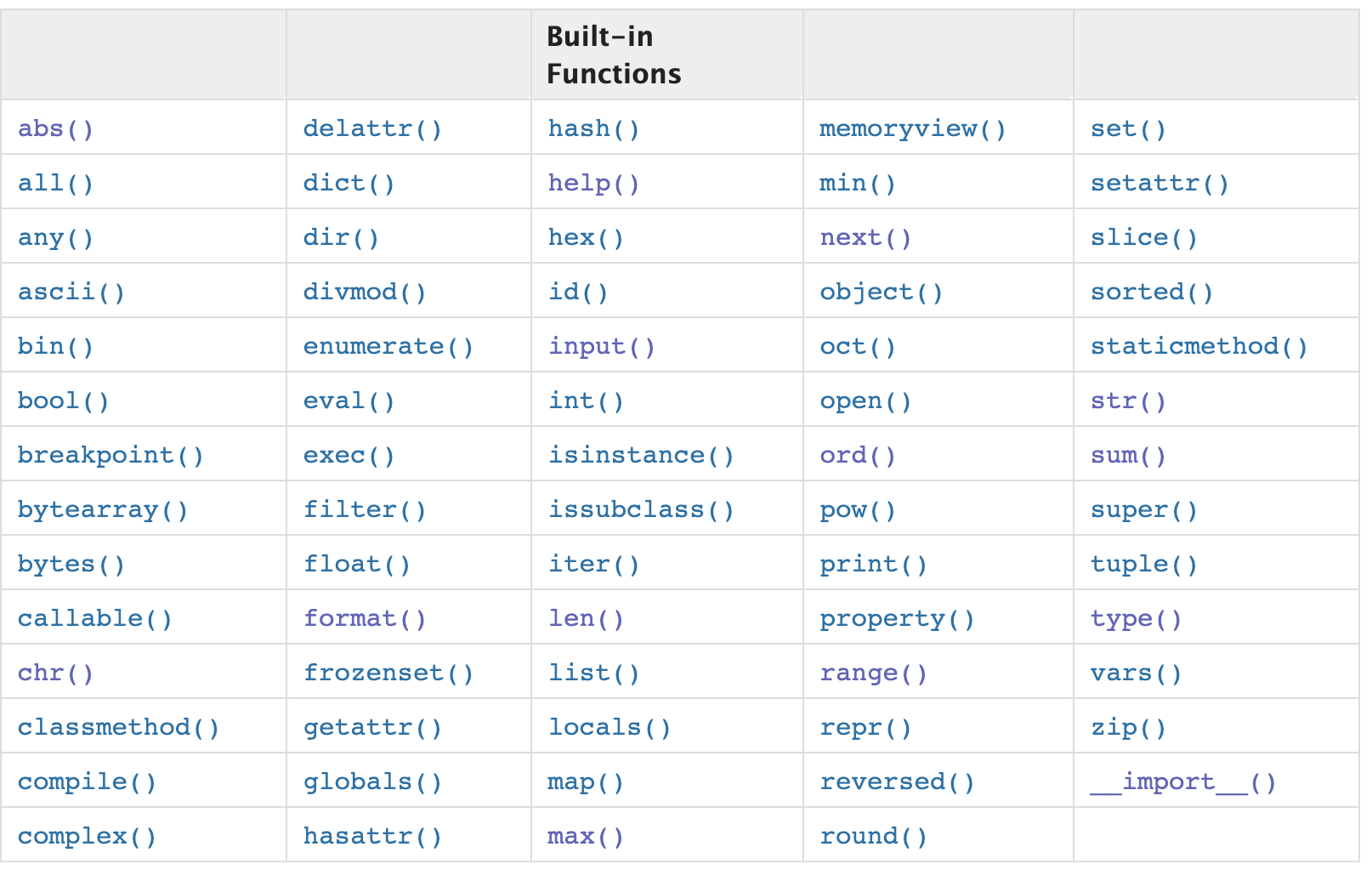## 1.6 Documentation and getting help

The comment character, # tells python to ignore everything written to the right of the #. We use the # for each line we would use for commenting our code. In jupyter notebooks the color of the text will change to indicate that you are writing a comment. In the example below, the commented text is in a different color from the code.

This is a single line comment:

Note the input and the output.

``print("Adding a single-line comment to our code")``
``Adding a single-line comment to our code``
``abs(-33) #this function computes the absolute value of -33``
``33``

You can also add many lines of “commented text” to your code using a triple set of double quotes around the text.

This is a multi-line comment:

``print("Adding a multi-line comment to our code")``
``Adding a multi-line comment to our code``
``"""This code is used to print a message of your choice. Notice that this message that is included in the triple double quotes will not execute."""``
``'This code is used to print a message of your choice. Notice that this message that is included in the triple double quotes will not execute.'``

Getting help and understand python’s semantics, syntax, Google Colab, and Jupyter ipython notebooks

There are several important resources you should familiarize yourself with:

1. The python language reference https://docs.python.org/3/reference/index.html#reference-index

This reference manual describes the syntax and “core semantics” of the language. It is terse, but attempts to be exact and complete. The semantics of non-essential built-in object types and of the built-in functions and modules are described in The Python Standard Library.

1. The python standard library https://docs.python.org/3/library/index.html#library-index

This library reference manual describes the standard library that is distributed with Python. It also describes some of the optional components that are commonly included in Python distributions.

1. help() function

The python help function is used to display the documentation of modules, functions, classes, keywords etc. If the help function is passed without an argument, then the interactive help utility starts up.

For example, if you wanted help about the `print` statement. Simple type:

``help("print")``

This will show the documentation related to how the print statement works.

2. Jupyter ipython notebooks

## 1.7 Summary

• Google Colaboratory is the space where you can plan and test your python programs
• ipython notebooks are a convenient medium for writing python code. Notebooks are saved as .ipynb files. The notebook type used in Google Colab are jupyter notebooks.
• You can begin coding in python by using it as a calculator.
• Basic arithmetic operators in python include `+`, `-`, `*`, `/`, `%`, `//`, and `**`.
• There are many pre-loaded functions available to act on python objects and manipulate data. Some examples include `abs()`, `max()`, `min()`, `print()`, and `round()`
• Comments are used to write text in English to document your work. There are two types of comments single line (preceded by the `#`) and multi-line comments (text is enclosed by triple quotes `"""..."""`)

## Exercise 1.1

1. What’s wrong with this code?

Provide an explanation and show the corrected code.

`min[(2,3,-4,5,6)]`

1. Display the following text to the screen:

`Hello. Welcome to my python program.`

## Exercise 1.2

1. Three MBA students purchased a different asset yesterday at the below listed price. Each sold their asset today at the listed selling price.

Calculate the percent return for each student rounded to two decimal points.

Jen \$25.50 \$40.20
Jacobi \$103.30 \$125.00
Mark \$86.02 \$91.41
1. Calculate the maximum of the LIST of returns

2. Divide 72 by 5 and find both the integer and the remainder

3. How would you code the minimum of prime numbers 0-10?

4. You go to the store with \$15 to buy pears, each pear is a \$1.35, how many pears can you buy? (You cannot buy partial pears so round your results).